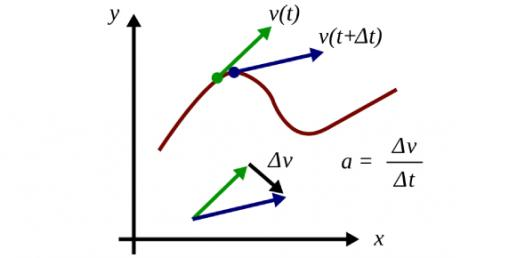# What Do You Know About Acceleration And Velocity? Trivia Quiz

10 Questions | Total Attempts: 15SettingsRelated Topics
• 1.
The units of acceleration are:-
• A.

Metres

• B.

Metres per second

• C.

Metres per second squared

• D.

Joules

• E.

Newtons

• 2.
A cyclist accelerates from rest to 12m/s in 4s. His acceleration is:-
• A.

48m/s2

• B.

3m/s2

• C.

0.33m/s2

• D.

1.33m/s2

• E.

0.75m/s2

• 3.
A car accelerates at 6m/s2 for 4s. If it starts at rest its final speed is:-
• A.

24m/s

• B.

1.5m/s

• C.

0.67m/s

• D.

9m/s

• E.

156m/s

• 4.
An aircraft on the runway accelerates at 8m/s2 from rest until it reaches its take-off speed of 60m/s. How long does it take to reach this speed?
• A.

68s

• B.

1.1s

• C.

480s

• D.

7.5s

• E.

48s

• 5.
A motor cyclist does an emergency stop when he is travelling at 36m/s. If the motorcycle stops in 3s what is his deceleration?
• A.

12m/s

• B.

108m/s

• C.

0.08m/s2

• D.

39m/s2

• E.

12m/s2

• 6.
A parachutist opens her parachute at an altitude of 1000m when she is travelling at 48m/s. The parachute causes her to decelerate at 4m/s2 for 8s. At what speed does she continue towards the ground?
• A.

32m/s

• B.

24m/s

• C.

12m/s

• D.

6m/s

• E.

1.5m/s

• 7.
To overtake a lorry while travelling at 15m/s a school minibus driver accelerates at 1.5m/s2 for 6s. What is the maximum speed the minibus achieves while overtaking?
• A.

22.5m/s

• B.

90m/s

• C.

81m/s

• D.

24m/s

• E.

32.5m/s

• 8.
A runner sprints for the line at the end of the 1500m. He is already running at 7.5m/s and he has worked out that he must be travelling at 9.3m/s in  less than 9s in order to win the race. What is the smallest acceleration that will allow him to do this?
• A.

0.2m/s2

• B.

1.1m/s2

• C.

1.87m/s2

• D.

1.8m/s2

• E.

45m/s2

• 9.
The key difference between velocity and speed is:-
• A.

Speed only applies to cars

• B.

Speed only applies when object move slowly.

• C.

Velocity is when the speed changes.

• D.

Velocity only applies to science experiments

• E.

Velocity is used when the direction matters.

• 10.
A car travelling at a constant speed around a roundabout is accelerating because:-
• A.

The car is slowing down

• B.

The car is speeding up

• C.

The car is not changing speed

• D.

The car is changing direction

• E.

The car is decelerating